Q-Chem 5.0 User’s Manual

# 3.3 Molecular Coordinate Input (\$molecule)

The \$molecule section communicates to the program the charge, spin multiplicity, and geometry of the molecule being considered. The molecular coordinates input begins with two integers: the net charge and the spin multiplicity of the molecule. The net charge must be between50 and 50, inclusive (0 for neutral molecules, 1 for cations,1 for anions, etc..). The multiplicity must be between 1 and 10, inclusive (1 for a singlet, 2 for a doublet, 3 for a triplet, etc..). Each subsequent line of the molecular coordinate input corresponds to a single atom in the molecule (or dummy atom), regardless of whether using Z-matrix internal coordinates or Cartesian coordinates.

Note: The coordinate system used for declaring an initial molecular geometry by default does not affect that used in a geometry optimization procedure. See Appendix A which discusses the Optimize package in further detail.

Q-Chem begins all calculations by rotating and translating the user-defined molecular geometry into a Standard Nuclear Orientation whereby the center of nuclear charge is placed at the origin. This is a standard feature of most quantum chemistry programs. This action can be turned off by using SYM_IGNORE TRUE.

Note:  SYM_IGNORE = TRUE will also turn off determining and using of the point group symmetry.

Note: Q-Chem ignores commas and equal signs, and requires all distances, positions and angles to be entered as ngstroms and degrees unless the INPUT_BOHR \$rem variable is set to TRUE, in which case all lengths are assumed to be in bohr.

## 3.3.1 Specifying the Molecular Coordinates Manually

### 3.3.1.1 Cartesian Coordinates

Q-Chem can accept a list of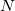atoms and their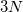Cartesian coordinates. The atoms can be entered either as atomic numbers or atomic symbols where each line corresponds to a single atom. The Q-Chem format for declaring a molecular geometry using Cartesian coordinates (in ngstroms) is:

```atom  x-coordinate  y-coordinate  z-coordinate
```

Note: The geometry can by specified in bohr by setting the \$rem variable INPUT_BOHR equal to TRUE.

Example 3.3  Atomic number Cartesian coordinate input for HO. The first line species the molecular charge and multiplicity, respectively.

```\$molecule
0 1
8   0.000000   0.000000  -0.212195
1   1.370265   0.000000   0.848778
1  -1.370265   0.000000   0.848778
\$end
```

Example 3.4  Atomic symbol Cartesian coordinate input for HO.

```\$molecule
0 1
O   0.000000   0.000000  -0.212195
H   1.370265   0.000000   0.848778
H  -1.370265   0.000000   0.848778
\$end
```

Note: (1) Atoms can be declared by either atomic number or symbol.
(2) Coordinates can be entered either as variables/parameters or real numbers.
(3) Variables/parameters can be declared in any order.
(4) A single blank line separates parameters from the atom declaration.

Once all the molecular Cartesian coordinates have been entered, terminate the molecular coordinate input with the \$end keyword.

### 3.3.1.2 Z-matrix Coordinates

For small molecules, Z-matrix notation is a common input format. The Z-matrix defines the positions of atoms relative to previously defined atoms using a length, an angle and a dihedral angle. Again, note that all bond lengths and angles must be in ngstroms and degrees, unless INPUT_BOHR is set to TRUE, in which case bond lengths are specified in bohr.

Note: As with the Cartesian coordinate input method, Q-Chem begins a calculation by taking the user-defined coordinates and translating and rotating them into a Standard Nuclear Orientation.

The first three atom entries of a Z-matrix are different from the subsequent entries. The first Z-matrix line declares a single atom. The second line of the Z-matrix input declares a second atom, refers to the first atom and gives the distance between them. The third line declares the third atom, refers to either the first or second atom, gives the distance between them, refers to the remaining atom and gives the angle between them. All subsequent entries begin with an atom declaration, a reference atom and a distance, a second reference atom and an angle, a third reference atom and a dihedral angle. This can be summarized as:

1. First atom.

2. Second atom, reference atom, distance.

3. Third atom, reference atom A, distance between A and the third atom, reference atom B, angle defined by atoms A, B and the third atom.

4. Fourth atom, reference atom A, distance, reference atom B, angle, reference atom C, dihedral angle (A, B, C and the fourth atom).

5. All subsequent atoms follow the same basic form as (4)

Example 3.5  Z-matrix for hydrogen peroxide

```  O1
O2   O1   oo
H1   O1   ho   O2   hoo
H2   O2   ho   O1   hoo   H1   hooh
```

Line 1 declares an oxygen atom (O1). Line 2 declares the second oxygen atom (O2), followed by a reference to the first atom (O1) and a distance between them denoted oo. Line 3 declares the first hydrogen atom (H1), indicates it is separated from the first oxygen atom (O1) by a distance HO and makes an angle with the second oxygen atom (O2) of hoo. Line 4 declares the fourth atom and the second hydrogen atom (H2), indicates it is separated from the second oxygen atom (O2) by a distance HO and makes an angle with the first oxygen atom (O1) of hoo and makes a dihedral angle with the first hydrogen atom (H1) of hooh.

Some further points to note are:

• Atoms can be declared by either atomic number or symbol.

• If declared by atomic number, connectivity needs to be indicated by Z-matrix line number.

• If declared by atomic symbol either number similar atoms (e.g., H1, H2, O1, O2 etc.) and refer connectivity using this symbol, or indicate connectivity by the line number of the referred atom.

• Bond lengths and angles can be entered either as variables/parameters or real numbers.

• Variables/parameters can be declared in any order.

• A single blank line separates parameters from the Z-matrix.

All the following examples are equivalent in the information forwarded to the Q-Chem program.

Example 3.6  Using parameters to define bond lengths and angles, and using numbered symbols to define atoms and indicate connectivity.

```\$molecule
0 1
O1
O2  O1  oo
H1  O1  ho  O2  hoo
H2  O2  ho  O1  hoo  H1  hooh

oo   =   1.5
oh   =   1.0
hoo  = 120.0
hooh = 180.0
\$end
```

Example 3.7  Not using parameters to define bond lengths and angles, and using numbered symbols to define atoms and indicate connectivity.

```\$molecule
0 1
O1
O2  O1  1.5
H1  O1  1.0  O2  120.0
H2  O2  1.0  O1  120.0  H1  180.0
\$end
```

Example 3.8  Using parameters to define bond lengths and angles, and referring to atom connectivities by line number.

```\$molecule
0 1
8
8  1  oo
1  1  ho  2  hoo
1  2  ho  1  hoo  3  hooh

oo   =   1.5
oh   =   1.0
hoo  = 120.0
hooh = 180.0
\$end
```

Example 3.9  Referring to atom connectivities by line number, and entering bond length and angles directly.

```\$molecule
0 1
8
8  1  1.5
1  1  1.0  2  120.0
1  2  1.0  1  120.0  3  180.0
\$end
```

Obviously, a number of the formats outlined above are less appealing to the eye and more difficult for us to interpret than the others, but each communicates exactly the same Z-matrix to the Q-Chem program.

### 3.3.1.3 Dummy Atoms

Dummy atoms are indicated by the identifier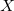and followed, if necessary, by an integer. (e.g.,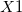,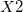. Dummy atoms are often useful for molecules where symmetry axes and planes are not centered on a real atom, and have also been useful in the past for choosing variables for structure optimization and introducing symmetry constraints.

Note: Dummy atoms play no role in the quantum mechanical calculation, and are used merely for convenience in specifying other atomic positions or geometric variables.

## 3.3.2 Reading Molecular Coordinates from a Previous Job or File

Often users wish to perform several calculations in sequence, where the later calculations rely on results obtained from the previous ones. For example, a geometry optimization at a low level of theory, followed by a vibrational analysis and then, perhaps, single-point energy at a higher level. Rather than having the user manually transfer the coordinates from the output of the optimization to the input file of a vibrational analysis or single point energy calculation, Q-Chem can transfer them directly from job to job.

To achieve this requires that:

• The READ variable is entered into the molecular coordinate input

• Scratch files from a previous calculation have been saved. These may be obtained explicitly by using the save option across multiple job runs as described below and in Chapter 2, or implicitly when running multiple calculations in one input file, as described in Section 3.6.

Example 3.10  Reading a geometry from a prior calculation.

```\$molecule
\$end
```

```localhost-1> qchem job1.in job1.out job1
localhost-2> qchem job2.in job2.out job1
```

In this example, the job1 scratch files are saved in a directory \$QCSCRATCH/job1 and are then made available to the job2 calculation.

Note: The program must be instructed to read specific scratch files by the input of job2.

The READ function can also be used to read molecular coordinates from a second input file. The format for the coordinates in the second file follows that for standard Q-Chem input, and must be delimited with the \$molecule and \$end keywords.

Example 3.11  Reading molecular coordinates from another file. filename may be given either as the full file path, or path relative to the working directory.

```\$molecule